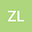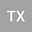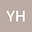Dynamic analysis of New Two Dimensional Fractional-order Discrete Chaotic Map and Its application in Cryptosystem
•••Tiecheng Xia
Shanghai University
Author ProfileYe Hu
Lvliang University
Author Profile## Abstract

A new fractional difference equation 2D-TFCDM based on Caputo derivative is proposed. Using the bifurcation diagram, the maximum Lyapunov exponent and the phase diagram, the numerical solutions of the fractional difference equations are obtained, and the chaotic behavior is observed numerically. After encrypting the key with elliptic curve cryptosystem, the fractional map is developed as an encryption algorithm and applied to color image encryption. Finally, the proposed encryption system is systematically analyzed from five main aspects, and the results show that the proposed encryption system has a good encryption effect. In respective of application, we apply the proposed discrete fractional map into color image encryption with the secret keys ciphered by Menezes-Vanstone Elliptic Curve Cryptosystem (MVECC). Finally, the image encryption algorithm is analysed in 4 aspects that indicates the proposed algorithm is superior to others.
29 Aug 2022Submitted to Mathematical Methods in the Applied Sciences
30 Aug 2022Submission Checks Completed
30 Aug 2022Assigned to Editor
02 Sep 2022Reviewer(s) Assigned
03 Sep 2022Review(s) Completed, Editorial Evaluation Pending
05 Sep 2022Editorial Decision: Revise Major